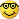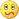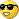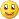ZigZag Channels! - page 4189

Also here's the USDJPY results for the last week ,1243

codersguru:
Very elegant chart XardYes the the default back is 0 but it should be 1. I'll check this in the next version and add alert when the price breaks the channel!

I was just wondering when you update your indicator if you could include a forward arc showing the settings that would trigger the next hi/low of the zigzag.

Xard777495

alert or line at next zigzag level

I agree with xard it would be very usefull if there where lines or something

where the next hi low zigzag

xard777:
I was just wondering when you update your indicator if you could include a forward arc showing the the settings that would trigger the next hi/low of the zigzag. Xard77712

Confius

Hai all ,

This is great zigzag , thank you Cg and Fx_sniper2 but can u Fx_Snipers2 explain to me want is small number at Extline example 2.24,1.414 and etc and how i want use this number for my guiding ?

Thank you5

Great stuff! Is it possible to also translate the Zig Zag Validity and Zig Zag Thresholds into MetaTrader?

Thanks5

ZigZag Validation, Trend, and Threshold

Here is the code for these three indicators written in metastock/tradestation language:

ZIG ZAG VALIDATION

This indicator validates the LAST LEG of Zigzag.

Values returned:

1 = valid leg (not revisable), 0 = invalid leg (revisable).

Do NOT use this indicator in systems or for backtesting.}

perc:=Input("Percent",0.0001,1000,10);

Z:=Zig(CLOSE,perc,%);

last:=ValueWhen(1,( Z > Ref(Z,-1) AND Ref(Z,-1) < Ref(Z,-2) )

OR

( Z Ref(Z,-2) ),

Ref(Z,-1));

pc:=(CLOSE-last) * 100 / last;

pc:= Abs(pc);

SD:=(z>Ref(z,-1) AND Ref(z,-1)>Ref(z,-2)) OR (z<Ref(z,-1) AND Ref(z,-

1)<Ref(z,-2));

res:=If(pc>=perc ,1,0);

ZIG ZAG TREND

{This is a safe indicator as it deals with valid trends only.

You can trade it directly or incorporate it in any other

tool or system.}

Values returned:

1 = Valid uptrend, -1 = valid downtrend }

vr:=Input("Field (0=Ind/tor, 1=Open, 2=High, 3=Low, 4=Close)",0,4,0);

amnt:=Input("Reversal amount",0.0001,1000,10);

md:=Input("Method (1=Percent, 2=Points)",1,2,1);

vr:=If(vr=1,OPEN,If(vr=2,HIGH,If(vr=3,LOW,If(vr=4, CLOSE,P))));

zz0:=If(md=1, Zig(vr,amnt,%), Zig(vr,amnt,\$));

zz1:=Ref(zz0,-1);

zz2:=Ref(zz0,-2);

tr:=ValueWhen(1,zz0>zz1 AND zz1<zz2, zz1);

pk:=ValueWhen(1,zz0zz2, zz1);

PU:=If(md=1,tr+Abs(tr)*amnt/100,tr+amnt);

PD:=If(md=1,pk-Abs(pk)*amnt/100,pk-amnt);

res:=If(vr>=PU AND zz0>zz1,1,

If(vr<=PD AND zz0<zz1,-1,0));

res:=If(res0,res,ValueWhen(1,res0,res));

res

ZIG ZAG THRESHOLD

{This indicator returns the price at which Zigzag Trend (ZZT) will change direction

(the price at which we will have a new confirmed trend)}

vr:=Input("Field (0=Ind/tor, 1=Open, 2=High, 3=Low, 4=Close)",0,4,0);

amnt:=Input("Reversal amount",0.0001,1000,10);

md:=Input("Method (1=Percent, 2=Points)",1,2,1);

vr:=If(vr=1,OPEN,If(vr=2,HIGH,If(vr=3,LOW,If(vr=4, CLOSE,P))));

zz0:=If(md=1, Zig(vr,amnt,%), Zig(vr,amnt,\$));

zz1:=Ref(zz0,-1);

zz2:=Ref(zz0,-2);

tr:=ValueWhen(1,zz0>zz1 AND zz1<zz2, zz1);

pk:=ValueWhen(1,zz0zz2, zz1);

PU:=If(md=1,tr+Abs(tr)*amnt/100,tr+amnt);

PD:=If(md=1,pk-Abs(pk)*amnt/100,pk-amnt);

res:=If(vr>=PU AND zz0>zz1,1,

If(vr<=PD AND zz0<zz1,-1,0));

res:=If(res0,res,ValueWhen(1,res0,res));

top:=HighestSince(1,res>Ref(res,-1),vr);

bot:=LowestSince(1,res<Ref(res,-1),vr);

threshold:=If(res=-1,

If(md=1,bot+Abs(bot)*amnt/100,bot+amnt),If(md=1,top-Abs(top)*amnt/100,top-amnt));

threshold;

Thanks!5

This is just what I was looking for

Hey CodesGuru, thanks a million! This is EXACTLY what I was looking for.

I'm creating an EA that trades trend-line breaks and uses the BrainTrading1Stop as a trailing stop loss.

Again, thanks allot!

Yam Digger3203

increase:
The problem with the zigzags seems to be the repainting, if this member reckons this does not repaint, couldnt someone fix it so it displays the zigzags? https://www.mql5.com/en/forum/176034

just interesting observation - doesn't seem like anyone worries about Price Cannel "repainting" (or Ichimoku [midlinesHH-LL/2]) and if ZZ- line from top to bottom of Price Cannel... we can leave the old ('counceled') leg and build new (two or three tops) - no repainting ... would it help?

- if there is special thread 4this - please, throw the link81

Hello! A very nice indicator, but I don't understand how to use it in expert advisor? I've tried this kind of code:

H1ZigZagUp = iCustom("GBPUSD",60,"ZigZag_Channels",12,5,3,Yellow,Yellow,1,2,0);

H1ZigZagBottom = iCustom("GBPUSD",60,"ZigZag_Channels",12,5,3,Yellow,Yellow,1,3,0);

But when i tested this, trend lines didn't change, they just stuck in their start position.

Does anybody know the proper solution?1259

Zz

Hi,

I think there's zigzag indicator that not repaint....HiLow Zigzag is not repaint===================

Forex Indicators Collection